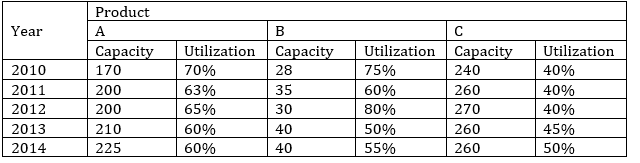Quantitative Aptitude Quiz For NIACL AO

Numerical Ability or Quantitative Aptitude Section has given heebie-jeebies to the aspirants when they appear for a banking examination. As the level of every other section is only getting complex and convoluted, there is no doubt that this section, too, makes your blood run cold. The questions asked in this section are calculative and very time-consuming. But once dealt with proper strategy, speed, and accuracy, this section can get you the maximum marks in the examination. Following is the Quantitative Aptitude quiz to help you practice with the best of latest pattern questions

Q1. The sum of four numbers is 64. If you add 3 to the first number, 3 is subtracted from the second number, the third is multiplied by 3 and the fourth is divided by three, then all the results are equal. What is the difference between the largest and the smallest of the original numbers?
32
27
21
Cannot be determined
None of these

Q2. Ms. Pooja Pushpan invests 13% of her monthly salary, i.e. Rs. 8554 in mediclaim Policies. Later she invests 23% of her monthly salary on Child Education Policies. Also, she invests another 8% in her monthly Funds. What is the total annual amount invested by Ms. Pooja Pushpan?
Rs. 28952
Rs. 43428
Rs. 347424
Rs. 173712
None of these

Q3. The batting average of 40 innings of a cricket player is 50 runs. His highest score exceeds his lowest score by 172 runs. If these two innings are excluded the average of the remaining 38 innings is 48. His highest score was:
172
173
174
176
None of these

Q4. A vessel contains liquid P and Q in the ratio 5 : 3. If 16 L of the mixture are removed and the same quantity of liquid Q is added, the ratio become 3 : 5. What quantity does the vessel hold?
35 L
45 L
40 L
50 L
None of these

Q5. Several litres of acid were drawn off a 54 L vessel full of acid and an equal amount of water added. Again, the same volume of the mixture 2 was drawn off and replaced by water. As a result the vessel contained 24 L of pure acid. How much of the acid was drawn off initially?
12 L
16 L
18 L
24 L
None of these

Directions (6-10): Find out the approximate value which should replace the question mark (?) in the following questions. (You are not expected to find out the exact value)

Q6. 459% of 849.947 + 266.5% of 6284.012 – 1486.002 = ?

20330
12640
15000
22160
19170

Q7. 21.0091 – 6.085 + 13.24 = (3.5 + ?) × 2
24.5
15.5
6.5
20.5
10.5

Q8.98
102
65
79
35

Q9. 1702 ÷ 68 × 136.05 = 50 × ?
80
74
77
68
63

Q10. 69.99% of 1401-13.99% of 1299=?
700
720
770
800
740

Directions (11-15): The table below gives the production capacity (in thousand units) and the percent utilization in respect of three products (A, B and C) over five years for an organization. Study the table carefully and answer the questions that follow.Q11. Find the average utilization of product C over the given years.

11100
101000
111000
120000
None of these

Q12. Total production capacity of three products in 2010 is by what percent more or less than total utilization in year 2014 ?
50.2%
52.6%
68.8%
60.2%
58.5%

Q13. What is the difference in the total production of A in 2010 and 2011 together and utilization of C in 2012, 2013 and 2014 together?
15500
14500
16000
14505
None of these

Q14. If in year 2013, all the unsatisfied products are recycled and reproduced in some quantity and among them 80% were utilized, then find the unutilized products at last.
44,800
49,400
44,900
49,000
None of these

Q15. Find the ratio of sum of capacity of A and C in 2011 and difference of the utilization of A and C in 2013.
460 : 11
360 : 91
406 : 9
306 : 11
460 : 9

a

You May also like to Read: